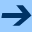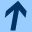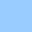Functional equations and iteration theory

## Functional equations and iteration theory

Functional equations and iteration theory is a scientific project supported by the Fonds zur Förderung der wissenschaftlichen Forschung. It has the project number P14342 - MAT. H. Fripertinger works in this project under the supervision of Prof. L. Reich of the Institut für Mathematik at the university of Graz.

During the last two or three decades many problems connected both with iteration theory and functional equations were studied. In some of these problems functional equations play a prominent, independent role in others they are just a tool for finding the solution. In addition to this it is interesting to apply results from iteration theory to functional equations. For doing this many different mathematical structures and methods are used.

In this project we propse the study of several problems from the theory of functional equations, in particular about functional equations in iteration theory. The research of members of the Institute of Mathematics of the Karl-Franzens University in Graz through many years indicates that these problems are interesting and promising. Besides iteration theory in rings of formal power series the sources of these problems are applications, algebraic aspects of additive functions, commutable functions and functional equations of iterative type.